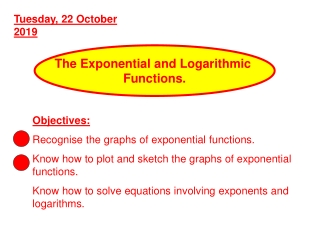DownloadDownload PresentationThe Exponential and Logarithmic Functions.

# The Exponential and Logarithmic Functions.

Télécharger la présentation## The Exponential and Logarithmic Functions.

- - - - - - - - - - - - - - - - - - - - - - - - - - - E N D - - - - - - - - - - - - - - - - - - - - - - - - - - -
##### Presentation Transcript

1. Tuesday, 22 October 2019 The Exponential and Logarithmic Functions. Objectives: Recognise the graphs of exponential functions. Know how to plot and sketch the graphs of exponential functions. Know how to solve equations involving exponents and logarithms.

2. Laws of Logarithms log a + log b = log ab Example: log 3 + log 5 = log 15 log a - log b = log (a/b) Example: log 21 – log 7 = log 3

3. Laws of Logarithms log a k = k loga Example: log 35 = 5 log 3

4. e = 2.718281828459045…... ‘the Base of Natural Logarithms’ x ( 1 + 1 ) It is defined as : lim x X The number e was first studied by the Swiss mathematician Leonhard Euler in the 1720s, although its existence was more or less implied in the work of John Napier, the inventor of logarithms, in 1614. Euler was also the first to use the letter e for it in 1727 (the fact that it is the first letter of his surname is coincidental). As a result, sometimes e is called the Euler Number, the Eulerian Number, or Napier's Constant (but not Euler's Constant). • An effective way to calculate the value of e is to use the following infinite sum: • e = 1/0! + 1/1! + 1/2! + 1/3! + 1/4! + ….

5. Why e ? e is a real number constant that appears in some kinds of mathematics problems. Examples of such problems are those involving growth or decay (including compound interest), the statistical "bell curve," the shape of a hanging cable (or the Gateway Arch in St. Louis), some problems of probability, some counting problems, and even the study of the distribution of prime numbers. It appears in Stirling's Formula for approximating factorials. It also shows up in calculus quite often, wherever you are dealing with either logarithmic or exponential functions. There is also a connection between e and complex numbers, via Euler's Equation. Also, you’ll soon see, it’s unique properties make it very powerful in calculus

6. y = ex When x=0 y = e0 = 1 So it goes through (0,1) The x-axis is an asymptote y = ex can, of course, be transformed

7. Natural Logarithms • Any log to the base e is known as a natural logarithm. • In French this is a logarithme naturel • Which is where ln comes from. • When you see ln (instead of log) • then it’s a natural log

8. y = ex y = ex When x=0 y = e0 = 1 So it goes through (0,1) The x-axis is an asymptote y = ex can, of course, be transformed

9. y = ex and y = x y = ex y = x

10. y = ex,y = x and y = ln x y = ex y = x y = ln (x) y=ln(x) is a reflection of y = ex in the line y = x y=ln(x) and y = ex are inverse functions

11. y = ex,y = x and y = ln x y = ex y > 0 y = x y = ln (x) Domain is x > 0

12. Sketching • y = e-x • y = 2ex • y = ln (x+1) • y = ln (3x) … naturally follows the laws of translations

13. y = ex and y = e-x andy = 2ex y = 2ex y = e-x y = ex y = e-x is a reflection in the y-axis y = 2ex scales by factor 2 in the y-direction

14. y = ex and y = ln (x+1)andy = ln 3x y = ln 3x y = ln (x+1) y = ln 3xscales by factor 3 in the y-direction y = ln (x+1)is a translation by -1 in the x-direction y = ln (x)

15. f(x) = ex+2 -5 f(x) = ex+2 - 5 a) f(-2) = e-2+2 - 5 f(-2) = e0 - 5 = 1 - 5 = -4

16. Sketch y = ex+2 -5 y = ex+2 (-2,1) (-2,-4) y = ex+2 - 5 y = ex (0,1) y = ex+2 is translation of -2 along the x-axis y = ex+2 - 5is translation of -5 along the y-axis f(-2) = -4

17. Sketch y = ex+2 -5 (-2,-4) y = ex+2 - 5 f(x) = ex+2 - 5 f(x) = 0 ex+2 - 5 = 0 ex+2 = 5 ln ex+2 =ln 5 x+2 = ln 5 x = ln 5 -2 = -0.39 (2 dp) f(0) = e2 - 5 = 2.4 (1 dp) (0, e2 - 5) (ln 5 -2, 0)